Mathematics

# What are Polygons?

0

 1 Introduction 2 What are Polygons? 3 Classification of polygons 4 Types of polygon 5 Area and perimeter of triangles 6 Area and perimeter of the pentagon 7 Area and perimeter of the hexagon 9 Summary 10 FAQs 11 External References

16 December 2020

Geometry is a branch of maths that deals with the study of various different shapes and their properties. When line segments join together to form a closed circuit then they are called "POLYGONS". Here we will study the properties of a few polygons.

## What are Polygons?

A closed GEOMETRICAL figure having a definite number of line segments connected together are called "POLYGONS"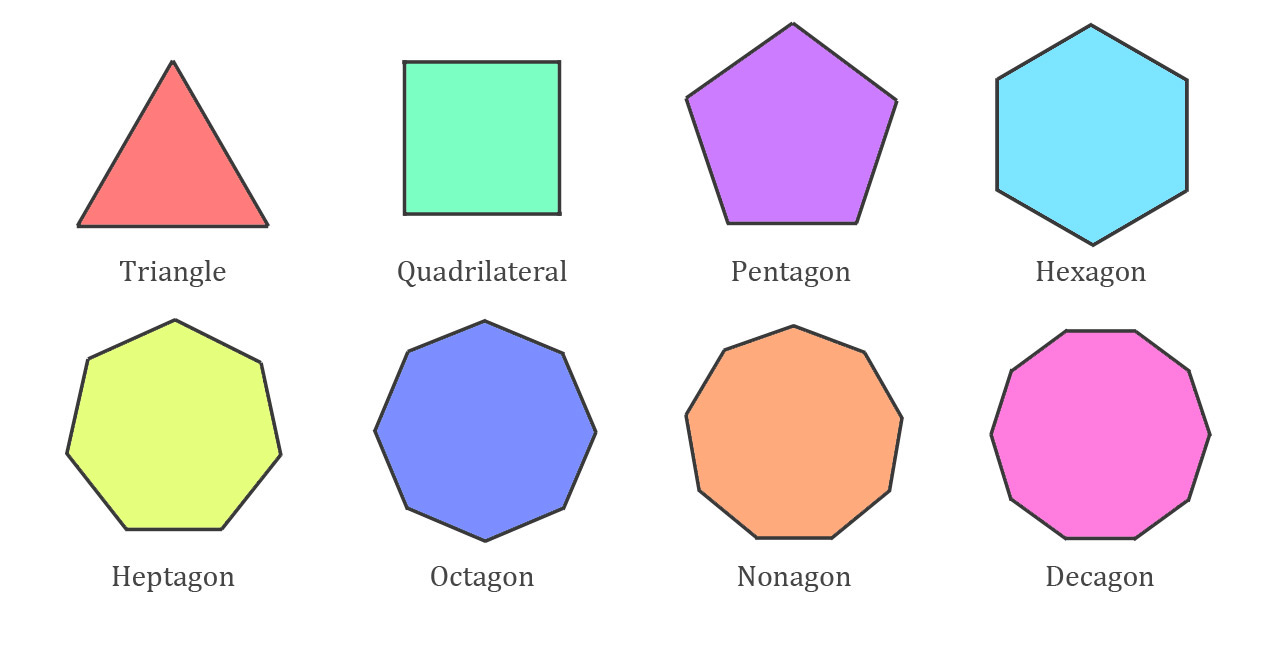Polygons can be broadly classified into four types depending on their shapes, they are:

1. Regular polygons
2. Irregular polygons
3. Concave polygons
4. Convex polygons

## What are Polygons? - PDF

If you ever want to read it again as many times as you want, here is a downloadable PDF to explore more.

## Classification of Polygons

Types of polygons Definition Examples
Regular polygons These are Polygons having the same length on all sides and having equal interior angles.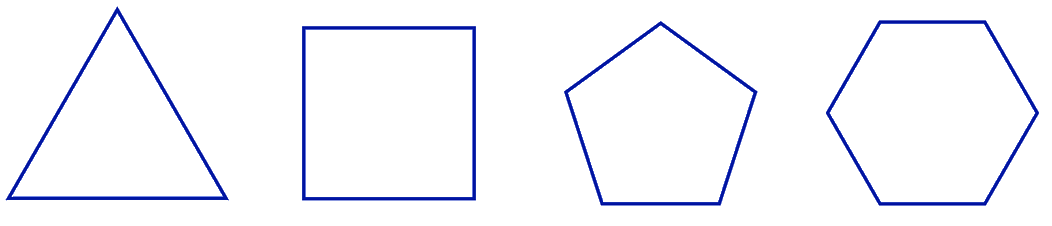Irregular polygons These Polygons do not have the same length on all sides and their interior angles also differ from each other.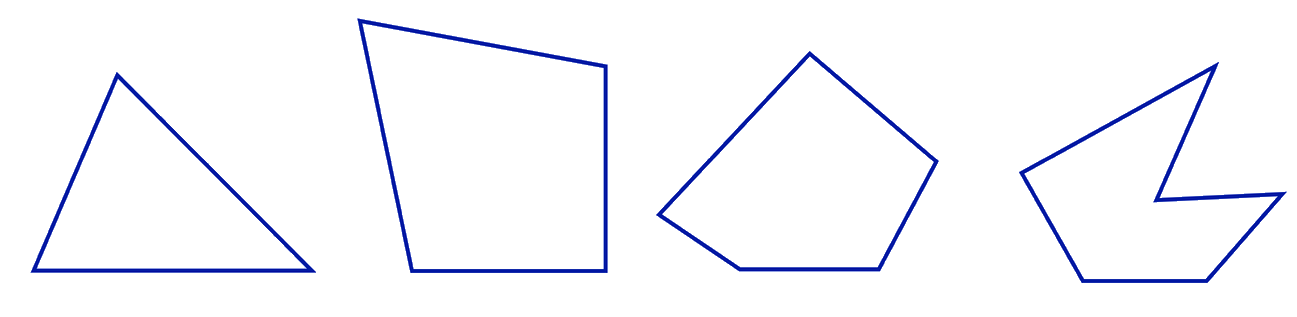Concave polygons If a polygon has one or more interior angles greater than 180 degrees then it is a concave polygon.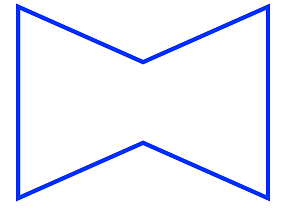Convex polygon These type of polygons have all their interior angle less than 180 degrees.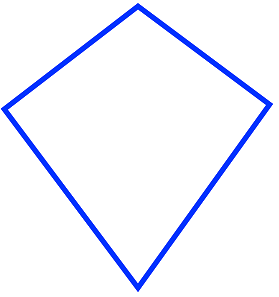## Different Types of Polygon

Type of Polygon Sides Shape Angles
Triangle 360o
Square 490o
Pentagon 5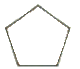108o
Hexagon 6120o
Heptagon 7128.5o
Octagon 8135o
Nonagon 9140o
Decagon 10144o

### No, circles are not polygons. A polygon is one that has a line segment connected to form a closed figure. But in the case of a circle, its sides intersect at any place other than at the end of its sides.

"The path that surrounds the area".

 Example 1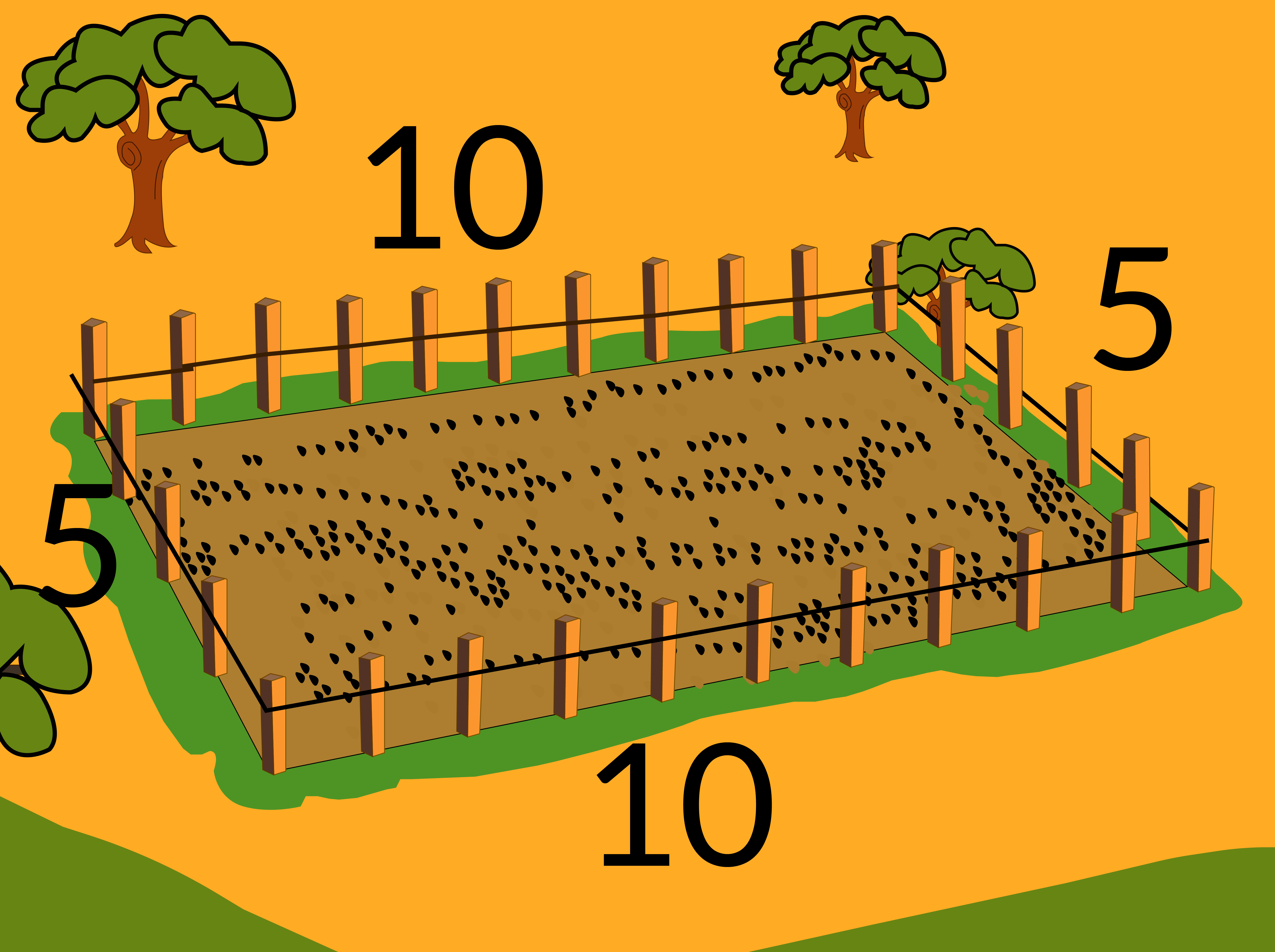Perimeter of above figure= 10+5+10+5=30 units.

In general, the Perimeter (P) of any polygon can be formulated by

 P=n x l where n = sides of the polygon l = length of their sides

The area of a polygon is the surface surrounded by a perimeter.

Area of the above figure, A=l x b (since it is a rectangle)

A=10 × 5 = 50 square units

Let's discuss in detail how to formulate the area and perimeter of the triangle, pentagon, and Hexagon?

## Area and perimeter of triangles

A triangle is a polygon having 3 sides and their interior angles summing up to 180°. Triangles can be classified into three types based on their sides and three types based on their angles.

The perimeter of a triangle = 3l

where l= length of sides

3= number of sides of the polygon

1. ### Based on the sides

 Scalene Triangle Lengths of all the sides of a triangle are not equal and their angles are different.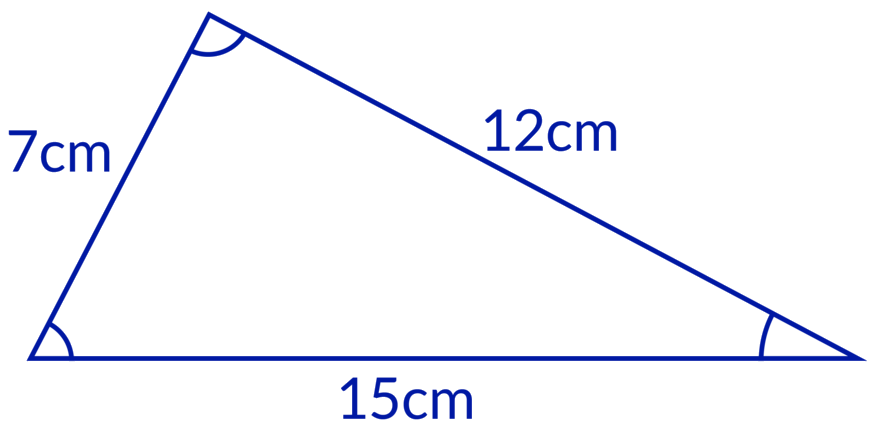Isosceles Triangle Any two side lengths and angles of a triangle are equal.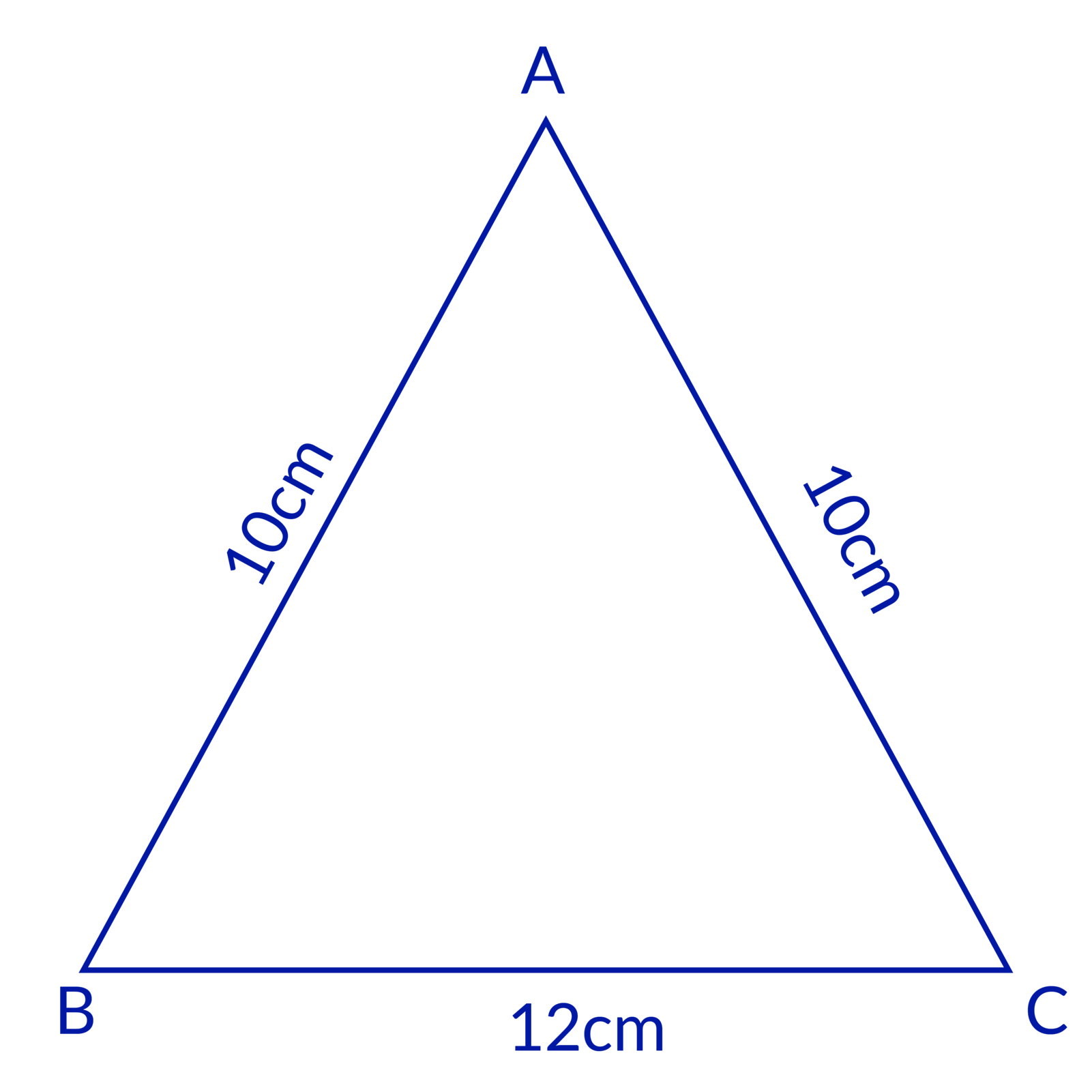Equilateral Triangle The length of all three sides and angles are equal.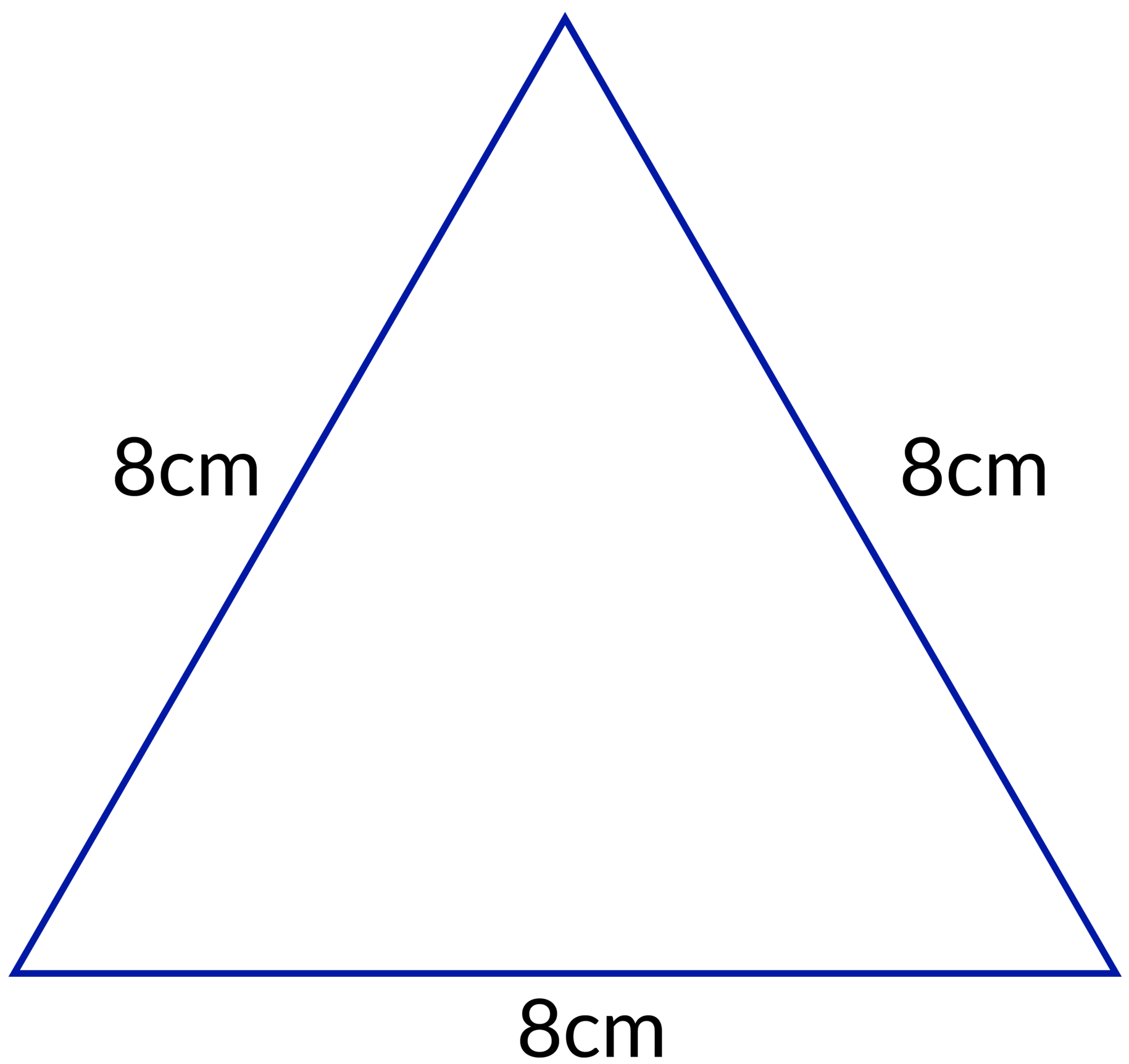1. ### Based on angle{interior angles} measurement

 Acute angled Triangle Angles measuring less than 90°.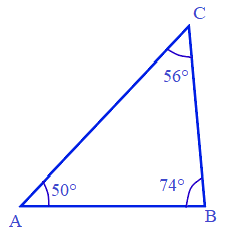Obtuse angled Triangle One of its angle measure more than 90°.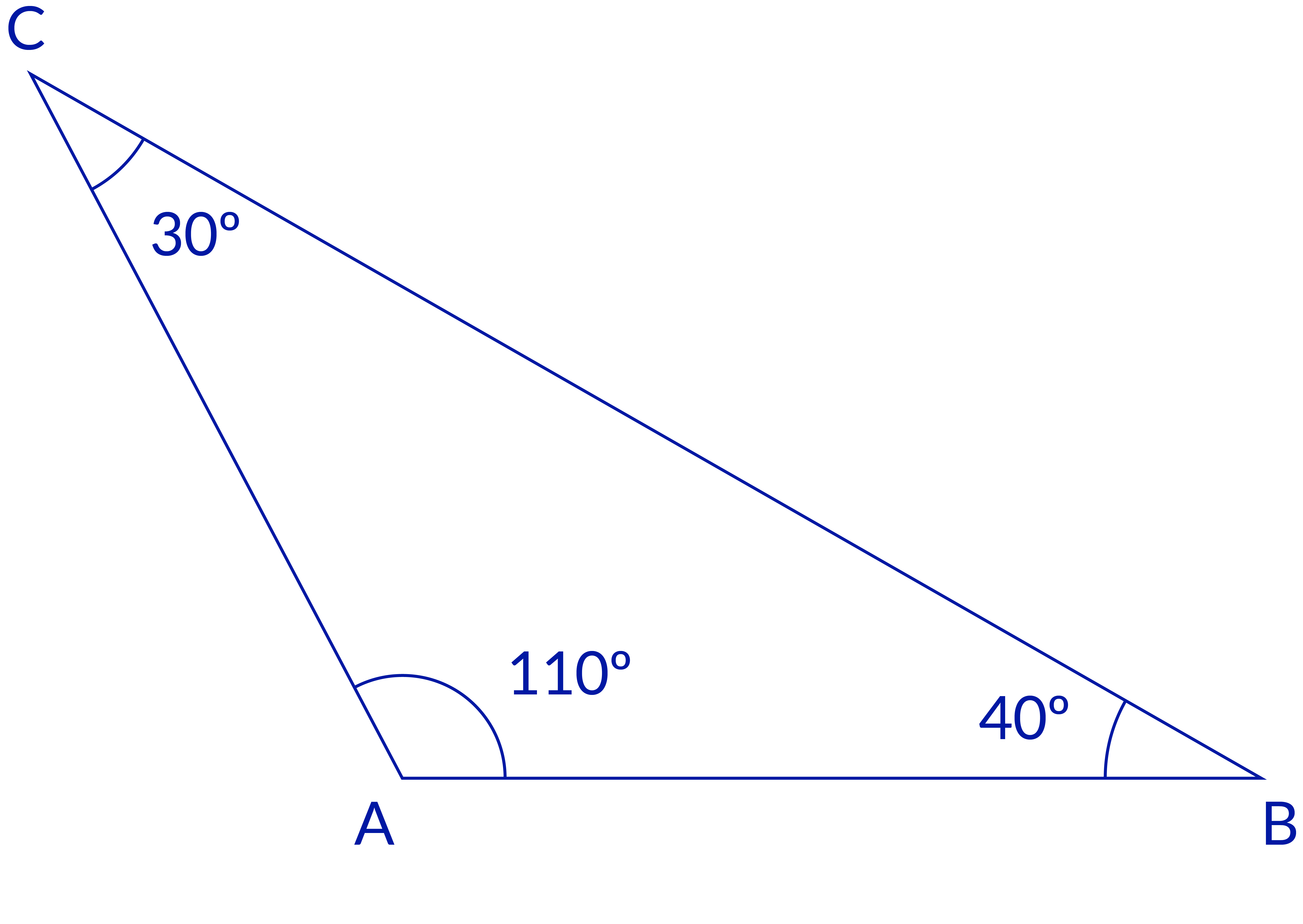Right-angled Triangle One of its angles measures 90° and the remaining two measures 45°.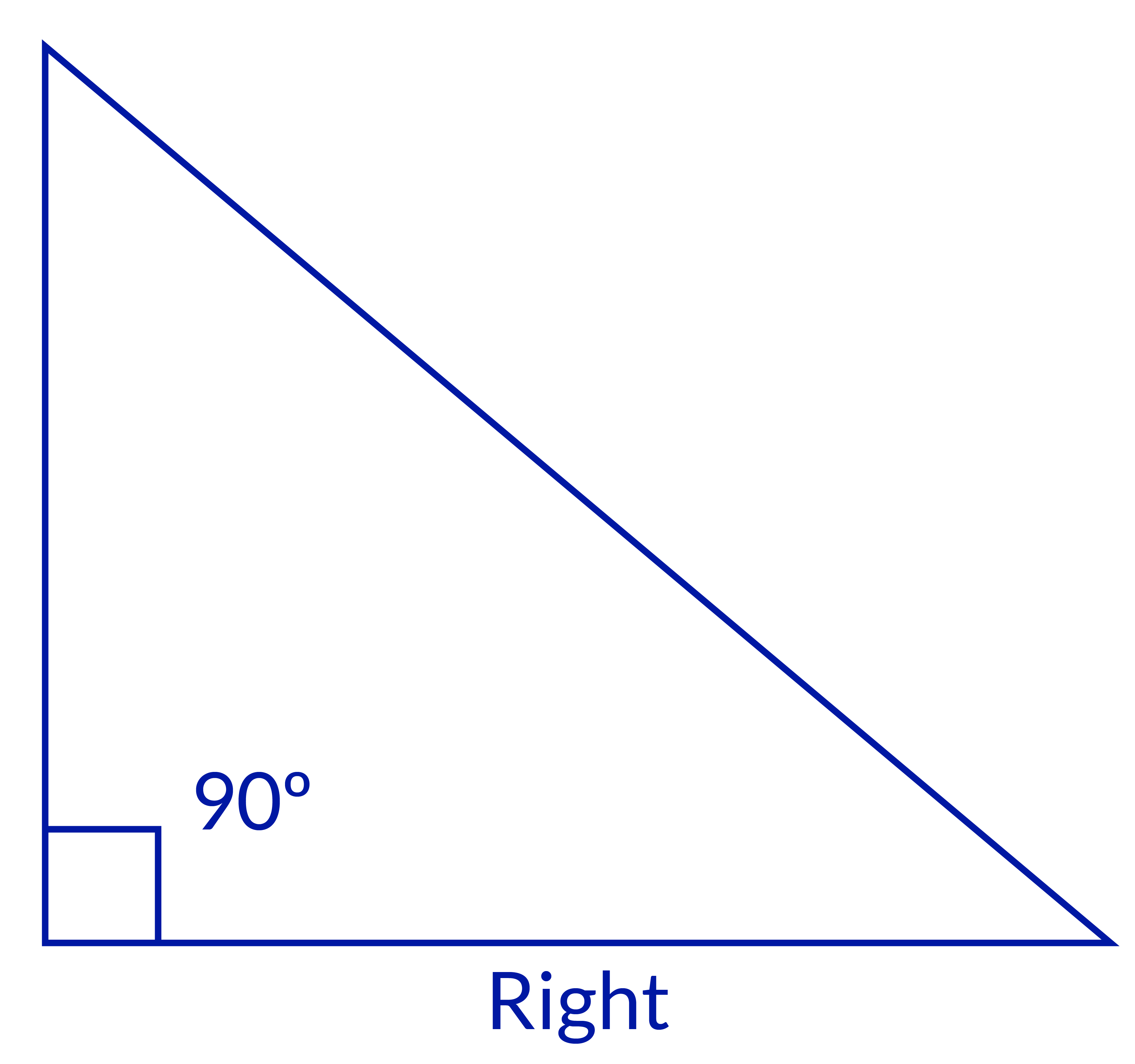### Area of a triangle

In general, the area of a triangle is defined as "half a time product of its base and height".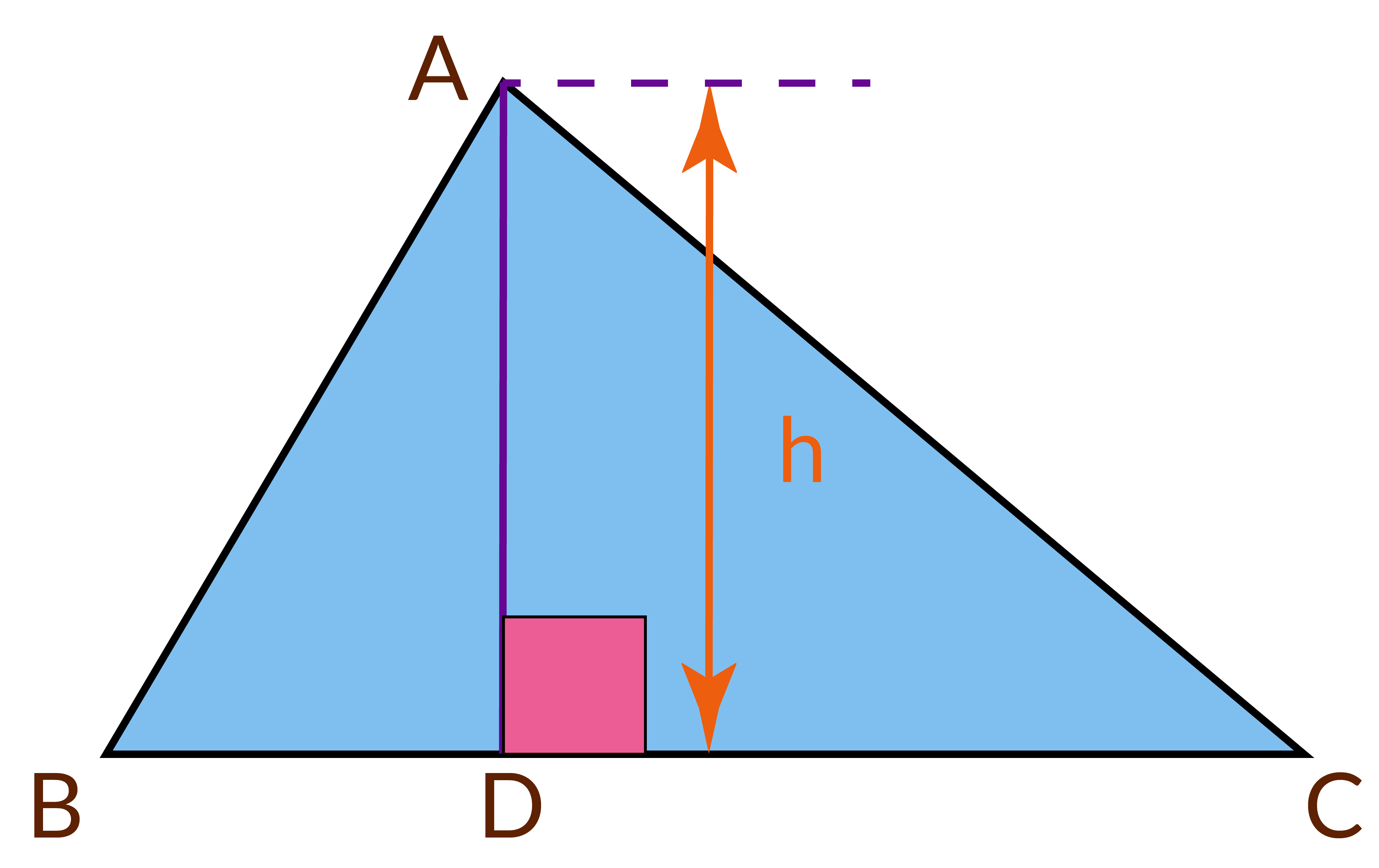A=½ x b x h Where b=base of the triangle h=height of the triangle.

### Formula to find Area of Equilateral Triangle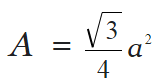### Formula to find the area of right-angled Triangle

 Area (A) =½ (b × c)

### Heron's Formula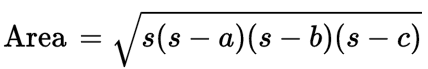where a, b, c are sides of the triangle s=semi perimeter
 Example 2

The sides of a triangular park are in the ratio 12:17:25 and its perimeter is 1080 m. What is its area?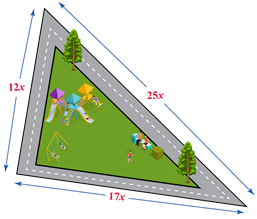Solution:

The sides of the triangle are 12x, 17x, and 25x.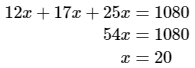Thus, the sides of the triangle are 240 m, 340 m, and 500m.

Now, the semi-perimeter of the triangle is, s=1080/2=540 m.

the area of the triangle is: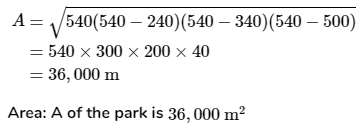## Area and perimeter of a Pentagon

Pentagon is a polygon having five sides and again Pentagon's may be regular, irregular, concave, and convex depending on sides. But, a regular pentagon has five sides of equal length and interior angle measuring 108° and an exterior angle of 72°.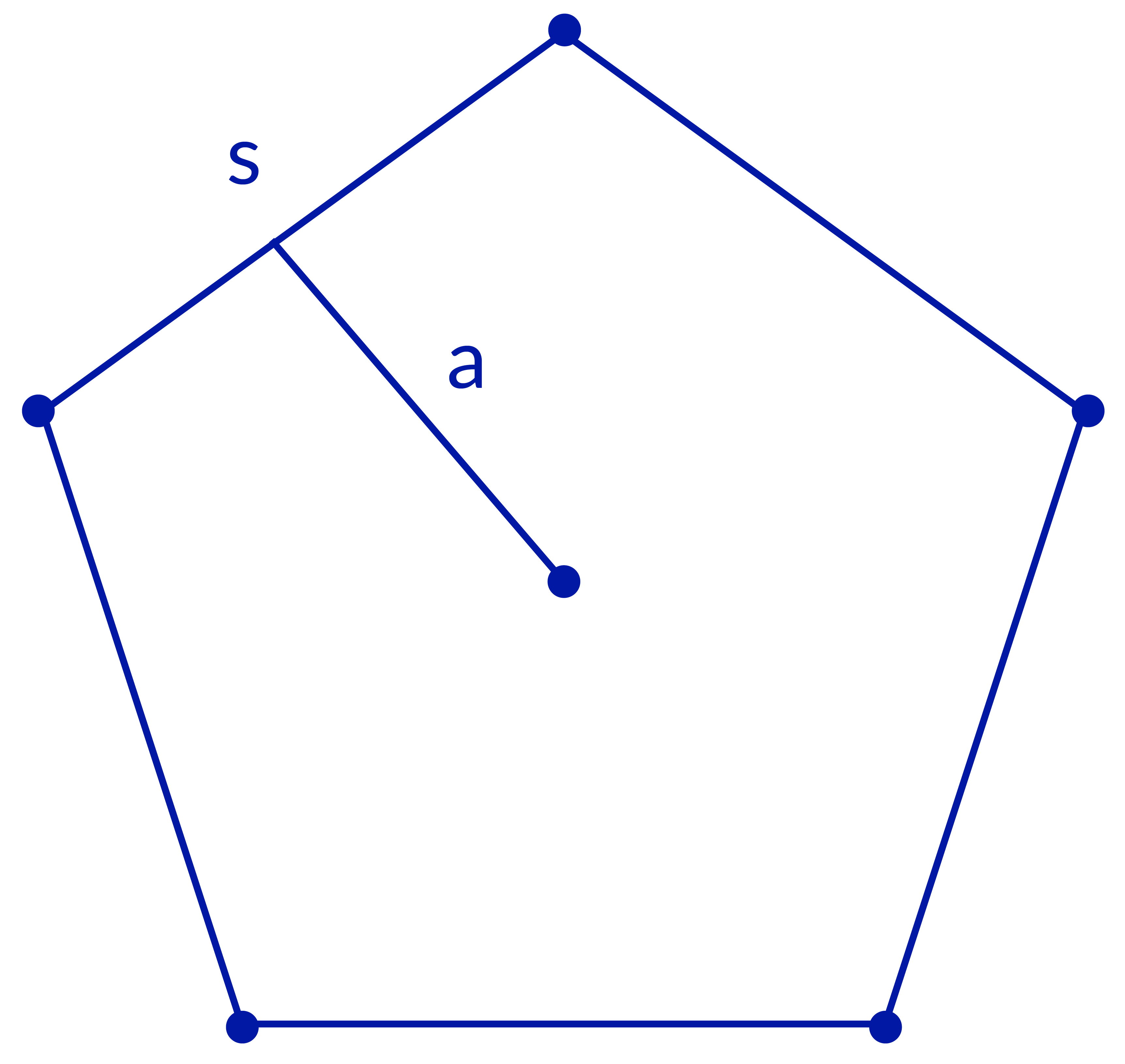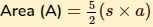Where s: side length of pentagon a: apothem length

The line segment drawn from the Centre of the pentagon to an is the side of the pentagon is called as "APOTHEM". It is calculated using the length of one side and the measure of the interior angle.

If only the side length of the pentagon is given: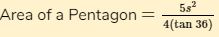Perimeter of pentagon (P) $$=5 × s$$ Where s =side length of pentagon.
 Example 3

Find the area and perimeter of a regular pentagon whose side is 7 cm and apothem length is 8 cm.

Solution:

Given: The side of a pentagon, a= 7cm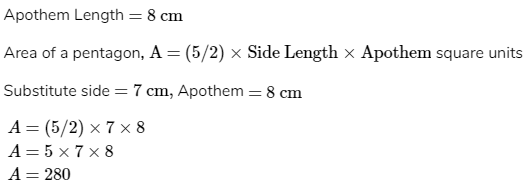Area = 280 sq.cm

Perimeter of a pentagon,

P = 5a units

P = 5(5)

P = 25 cm

 Perimeter = 25 cm.

## Area and perimeter of Hexagon

A hexagon is a polygon having 6 sides and when divided into a triangle they form 6 Equilateral triangles it has an interior angle of 120° and an exterior angle of 60°.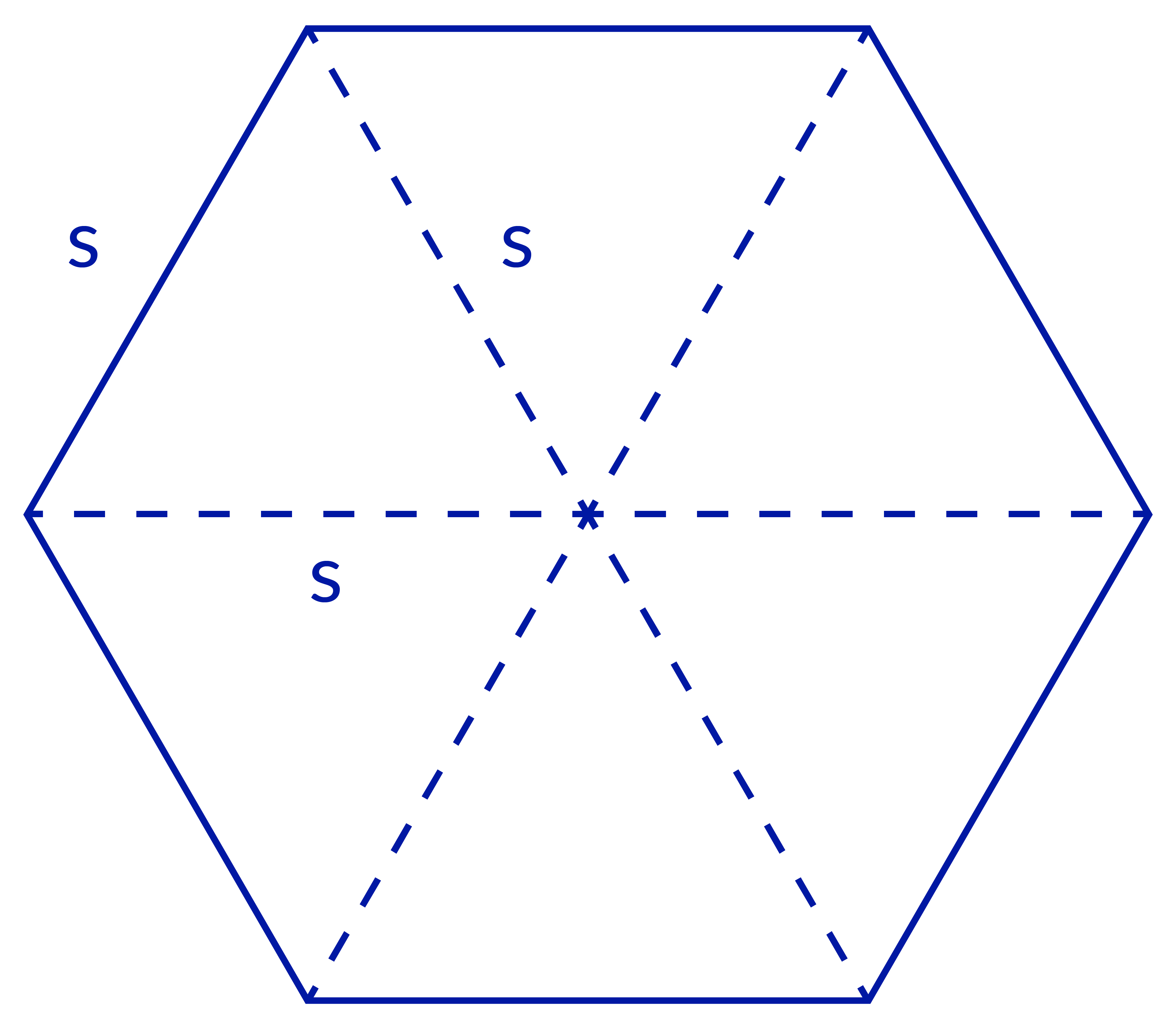Perimeter P = 6 x s Where, s = side length of Hexagon.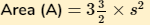Example 4

If the area of the hexagon is 25 sq.cm. Find its perimeter.

Solution:

Given, Area A = 25 sq.cm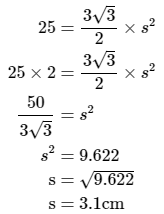Therefore the side length of the hexagon is 3.1 cm.

Perimeter=number of sides×side length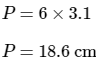## Summary

From the above topic, we clearly understood the properties of a few polygons and how to formulate their area and perimeter. A closed geometrical figure having a definite number of line segments connected together are called Polygons. They are of different types and have different angles and properties.

Cuemath is an online program that completely focuses on the holistic growth of children in math. Cuemath also offers a coding program for young coders. To learn math in a practical way and apply the concept in daily life please feel free to book your demo class.

Cuemath, a student-friendly mathematics and coding platform, conducts regular Online Live Classes for academics and skill-development, and their Mental Math App, on both iOS and Android, is a one-stop solution for kids to develop multiple skills. Understand the Cuemath Fee structure and sign up for a free trial.

## How many sides does a pentagon have?

A Pentagon is a 5 sided polygon.

## How to find the area of a hexagon?

The Area of a Hexagon can be expressed as 6 times the area of an equilateral triangle, which is written as $$\frac{3\sqrt{3}}{2}\times a^2$$

## How many sides does a hexagon have?

A Hexagon has 6 sides.

## How many diagonals does a hexagon have?

A Hexagon has 9 diagonals.

## External References

Written by Nethravathi C, Cuemath Teacher

GIVE YOUR CHILD THE CUEMATH EDGE
Access Personalised Math learning through interactive worksheets, gamified concepts and grade-wise courses